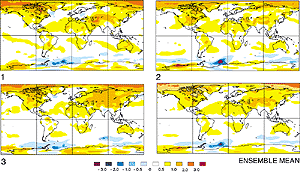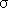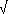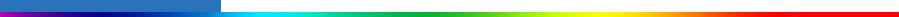Working Group I: The Scientific BasisGet Javascript Other reports in this collection

9.2.2 Simulating Forced Climate Change

#### 9.2.2.1 Signal versus noiseFigure 9.2: Three realisations of the geographical distribution of temperature differences from 1975 to 1995 to the first decade in the 21st century made with the same model (CCCma CGCM1) and the same IS92a greenhouse gas and aerosol forcing but with slightly different initial conditions a century earlier. The ensemble mean is the average of the three realisations. (Unit: °C).

A climate change simulation produces a time evolving three dimensional distribution of temperature and other climate variables. For the real system or for a model, and taking temperature as an example, this is expressed as T = T0 + T0' for pre-industrial equilibrium conditions. T is now the full temperature field rather than the global mean temperature change of Section 9.2.1. T0 represents the temperature structure of the mean climate, which is determined by the (pre-industrial) forcing, and T0' the internally generated random natural variability with zero mean. For climate which is changing as a consequence of increasing atmospheric greenhouse gas concentrations or other forcing changes, T = T0 + Tf + T' where Tf is the deterministic climate change caused by the changing forcing, and T' is the natural variability under these changing conditions. Changes in the statistics of the natural variability, that is in the statistics of T0' vs T', are of considerable interest and are discussed in Sections 9.3.5 and 9.3.6 which treat changes in variability and extremes.

The difference in temperature between the control and climate change simulations is written asT = Tf + (T' - T0') = Tf + T'', and is a combination of the deterministic signal Tf and a random component T'' = T' - T0' which has contributions from the natural variability of both simulations. A similar expression arises when calculating climate change as the difference between an earlier and a later period in the observations or a simulation. Observed and simulated climate change are the sum of the forced �signal� and the natural variability �noise� and it is important to be able to separate the two. The natural variability that obscures the forced signal may be at least partially reduced by averaging.

#### 9.2.2.2 Ensembles and averaging

An ensemble consists of a number of simulations undertaken with the same forcing scenario, so that the forced change Tf is the same for each, but where small perturbations to remote initial conditions result in internally generated climate variability that is different for each ensemble member. Small ensembles of simulations have been performed with a number of models as indicated in the �number of simulations� column in Table 9.1. Averaging over the ensemble of results, indicated by braces, gives the ensemble mean climate change as {T} = Tf + {T''}. For independent realisations, the natural variability noise is reduced by the ensemble averaging (averaging to zero for a large enough ensemble) so that {T} is an improved estimate of the model�s forced climate change Tf. This is illustrated in Figure 9.2, which shows the simulated temperature differences from 1975 to 1995 to the first decade in the 21st century for three climate change simulations made with the same model and the same forcing scenario but starting from slightly different initial conditions more than a century earlier. The differences between the simulations reflect differences in the natural variability. The ensemble average over the three realisations, also shown in the diagram, is an estimate of the model�s forced climate change where some of this natural variability has been averaged out.

The ensemble variance for a particular model, assuming there is no correlation between the forced component and the variability, is2T = {(T - {T})2} = {(T'' - {T''})2} =2N which gives a measure of the natural variability noise. The �signal to noise ratio�, {T}/T , compares the strength of the climate change signal to this natural variability noise. The signal stands out against the noise when and where this ratio is large. The signal will be better represented by the ensemble mean as the size of the ensemble grows and the noise is averaged out over more independent realisations. This is indicated by the width, {T} ± 2T /n, of the approximate 95% confidence interval which decreases as the ensemble size n increases.

The natural variability may be further reduced by averaging over more realisations, over longer time intervals, and by averaging in space, although averaging also affects the information content of the result. In what follows, the geographical distributionsT, zonal averages [T], and global averages <T> of temperature and other variables are discussed. As the amount of averaging increases, the climate change signal is better defined, since the noise is increasingly averaged out, but the geographical information content is reduced.